Diagrammatic Reasoning Practice Test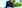# Diagrammatic Reasoning

Updated December 20, 2021

Diagrammatic reasoning questions (also known as logical reasoning or inductive reasoning questions) are designed to assess your logical reasoning ability. They consist of multiple-choice questions and are administered under exam conditions.

Many of the aptitude tests that you will face during the job selection process will contain questions of this type. These questions may either be integrated into a test along with verbal reasoning and numerical reasoning questions, or they may be presented as a separate diagrammatic reasoning test paper.

The aptitudes measured by questions that use words and numbers can easily be related to real jobs, which invariably require some degree of literacy and numeracy. Questions which use figures and diagrams seem to have very little application to regular professions and yet these types of question appear in most graduate and management aptitude tests. This is because evidence from psychology shows that this type of pure reasoning question is less dependent on your educational and cultural background than either verbal or numerical questions.

If you want to practise example tests and improve your performance, you can take practice diagrammatic reasoning tests here.

## What to Expect From Your Diagrammatic Reasoning Test

Diagrammatic reasoning tests are designed to be challenging, in order to differentiate between candidates and to identify the maximum performance they are capable of.

They usually have tight time scales, typically 30 minutes to complete 20 questions. and questions that rapidly increase in difficulty.

This means that you will need to identify more rules to solve the problems, and that the complexity of these rules is likely to increase.

While the various test providers use different question types and formats, there are some common general concepts that it is worth understanding. We'll cover these in the sections that follow.

## Practice Diagrammatic Reasoning Questions: An Example

Diagrammatic reasoning questions measure your ability to infer a set of rules from a flowchart or similar diagram, and then to apply those rules to a new situation. These questions are often found in tests aimed at selecting people who need to work through complex, and often conceptual, problems in an analytical way.

In this example, the diagram shows 'inputs' and 'outputs' in the large boxes. The 'operators' or 'processes' are shown in the small boxes. You need to determine what effect each of the 'operators' or 'processes' is having on the 'input' in order to produce the 'output' shown.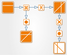Diagrammatic Reasoning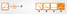Diagrammatic Reasoning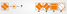Diagrammatic Reasoning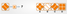Diagrammatic Reasoning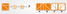Diagrammatic Reasoning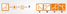Diagrammatic Reasoning

In this example, there are two operations separating each input from the output. The first task is to isolate the function of one of these operations and then to use a process of elimination to work out what each operator does.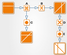Diagrammatic Reasoning

If we examine the Operator C/E in the diagram above, we can deduce that it cannot reverse the colours of the input because in Path A-C the input and output colours are the same.

Using this piece of information, we can assume that Operator D is reversing input and output colours. Looking at Path D/E, this means that Operator C/E must be reflecting the input in the vertical plane.

Using this piece of information, we can deduce that Operator A is adding the angled line to the input, from top left to bottom right.

Finally, we can deduce that Operator B must be rotating the input 90 degrees clockwise.

Now that we know what effect each of the operators has, we can proceed to answer the questions.

The answers in order therefore are: D, B, A, C, B. The original test is available here (20 minutes).

## Take a Free Practice Diagrammatic Test

If you would like to practise a simulation diagrammatic reasoning test, please try the one below, which was created by JobTestPrep in association with psychometric experts, and is closely modelled on real tests.

This test is slightly harder than the real thing, in order to make it sufficiently challenging practice.

You need to get 80% correct (4 out of 5) to pass the test. Don't forget to first check out the test tips and techniques section further down this page.

You can take the test as many times as you like.

undefined

## What Is the Difference Between Diagrammatic Reasoning and Abstract Reasoning?

The term diagrammatic reasoning is often used interchangeably with abstract reasoning by employers, although technically speaking abstract reasoning questions are slightly different to diagrammatic reasoning questions.

For example, abstract reasoning questions tend to focus on working out new concepts and abstract ideas, and are more closely correlated with questions in IQ tests that assess a candidate's ability to see the underlying logic in a pattern of symbols or shapes. So you may be asked to complete a sequence, identify the odd one out, or say what relation A is to B, given C's relationship to D. To give you an idea, see the diagrams in our article on abstract reasoning questions.

Diagrammatic reasoning questions are a little different in that they might use letters and numbers (where abstract reasoning tends to be more based on shapes) and there will sometimes be operators and processors as part of the question (as in the sample question above). Both tests rely on a logical thought process to deduce patterns.

If you are told that the test you are going to sit contains diagrammatic reasoning questions, then it is a good idea to ask to see a sample of the types of question that it will contain. This will allow you to find out exactly what sort of questions you are going to be asked.

## Practice Abstract Reasoning Questions: Some Examples

The following questions give you an idea of how abstract reasoning works. Each question involve a series of figures which appear in a sequence or pattern – these can be thought of as the 'problem' figure. You need to analyse this problem figure and determine which of the answer figures best completes it.

The answer in each case is just below the diagram, so don't scroll down too far!

### Which Symbol in the Answer Figure Best Completes the Sequence in the Problem Figure?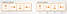Diagrammatic Reasoning

In this example, the question figure is rotated clockwise through 90 degrees each time.

The answer is therefore option A, which represents the last shape rotated through a further 90 degrees.

### Which of the Answer Figures Best Fits the Missing Space in the Question Figure?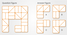Diagrammatic Reasoning

In this example, each complete row and column of the question figure contains one line of each type – horizontal, vertical, bottom-left-to-top-right and top-left-to-bottom-right. Option D is, therefore, the correct answer, as when this is used to complete the figure each row and column contains one of each line type.

## Tips and Best Techniques for Diagrammatic Reasoning Tests

These four tips are well worth remembering before you take the inductive reasoning test for real:

• Bring and use a stopwatch
• Learn the differences between test providers in terms of how they frame questions and how long the test will be
• Work swiftly but carefully
• Calculate the maximum amount of time to be allocated to a question and stick to it, using your stopwatch

The tests are timed, and typically there is time pressure. Most online tests are designed to be completed within the time allocated.

### Useful Techniques

Good exam technique for this type of question is to write down a note about the operation next to the symbol as you work them out. Often when performing written tests at assessment centres, you will not be allowed to write on the original question paper. In this case, you are usually provided with scrap paper onto which you can do a quick sketch. It is very easy to make mistakes on this type of question while under pressure, so make sure you take time and care to not become confused when answering - a single incorrect assumption can derail all your answers for the question.

It can be really helpful to practise some diagrammatic reasoning tests before you have to complete one under test conditions. This is because they are very different from the sorts of problems one tends to encounter in everyday life. Familiarity with the types of questions they include will help ensure that you don’t panic or freeze when you first see them.

Moreover, the more examples you work through, the more sorts of patterns you will encounter and the more of a ‘feel’ you will get for the materials. Make sure that you memorise the patterns discussed above but don’t let them blind you to creative alternative solutions.

Sometimes there may be questions where however much you look at them, you can’t see the pattern. Once you have given the question a good try move on. Don’t waste your time wrestling with one question at the expense of your overall performance.

You can find examples of each type of question at Job test Prep and AssessmentDay.